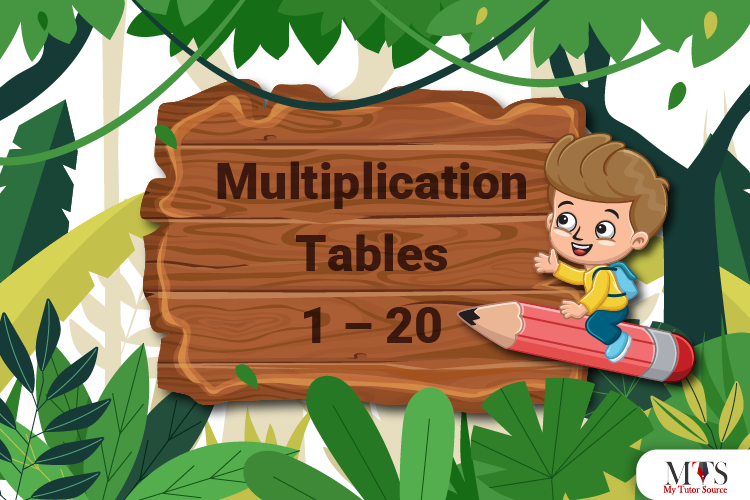## Multiplication Tables 1 – 20

Math multiplication tables are essential to skillfully and confidently solve mathematical questions quickly. No matter how rapidly the world changes, the traditional method of memorizing…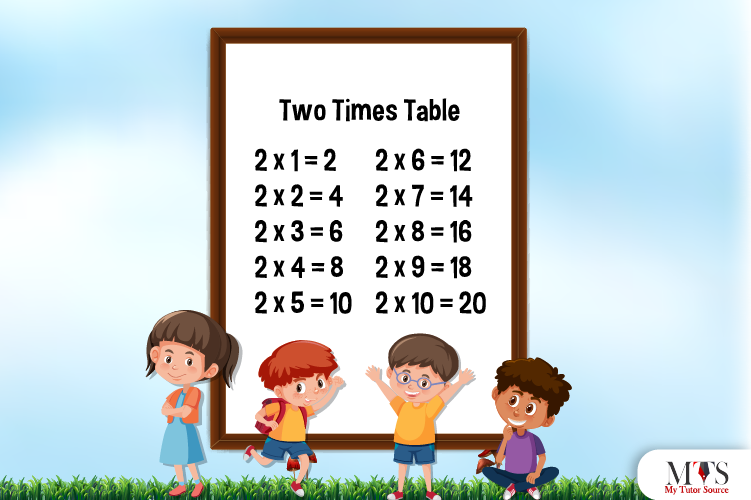## Multiplication Table of 2 – Tips to Memorize 2 Times Table & Example Questions

Table of 2 is the first step to making mental arithmetic easier. Multiplication skills are crucial for understanding intimidating and complex problems of mathematics. Math…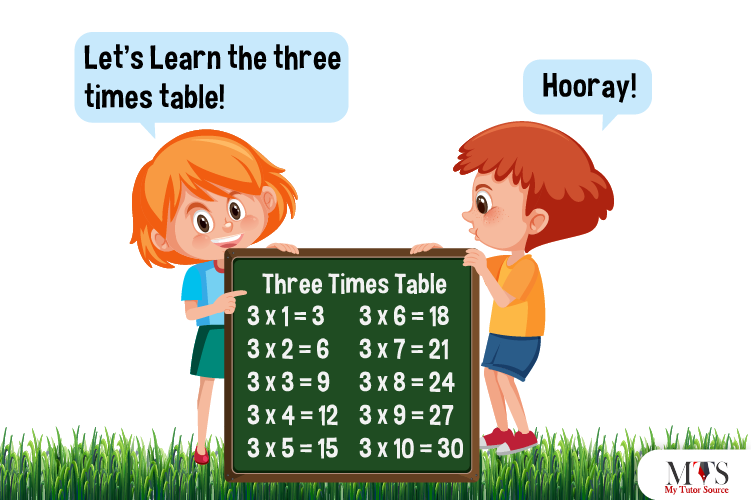## Multiplication Table of 3 – Tips to Memorize 3 Times Table & Example Questions

After memorizing the table of 2 on fingertips, the multiplication table of 3 would be less of a daunting task. Math comes with tricky and…## Multiplication Table of 4 – Tips to Memorize 4 Times Table & Example Questions

Time to learn the multiplication table of 4. Another even number multiplication table like 2 times table. According to professors and private math tutors, memorizing…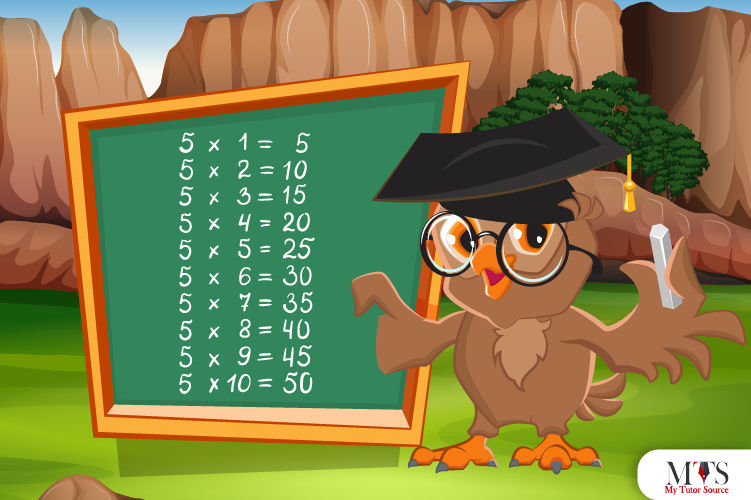## Multiplication Table of 5 – Tips to Memorize 5 Times Table & Example Questions

Learning lower times tables could be challenging for students, but 5 times table is a breeze. Math tutors and early elementary school teachers ensure their…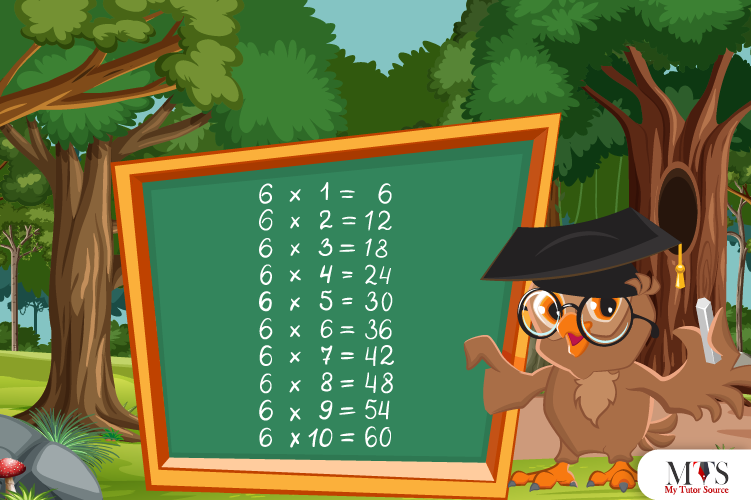## Multiplication Table of 6 – Tips to Memorize 6 Times Table & Example Questions

Learning multiplication tables are fundamental for everyday calculations. The table of 6 is the product of the number six with whole numbers. After the table…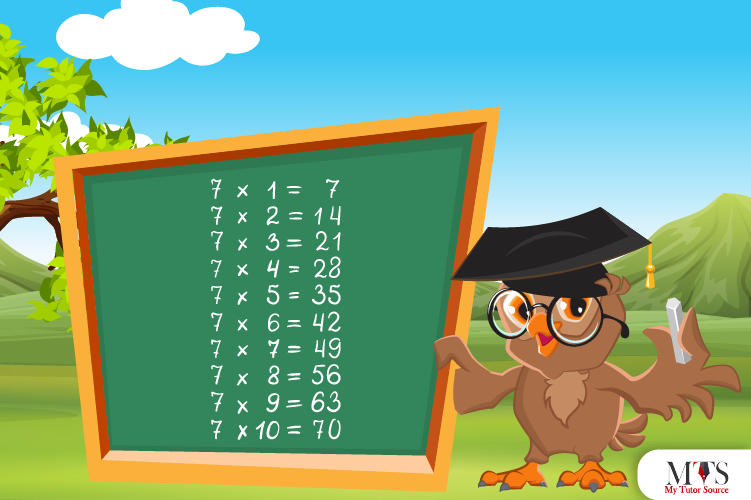## Multiplication Table of 7 – Tips to Memorize 7 Times Table & Example Questions

Save your time – learn multiplication tables to minimize the use of calculators during exams. 7 is the most used digit in our everyday life.…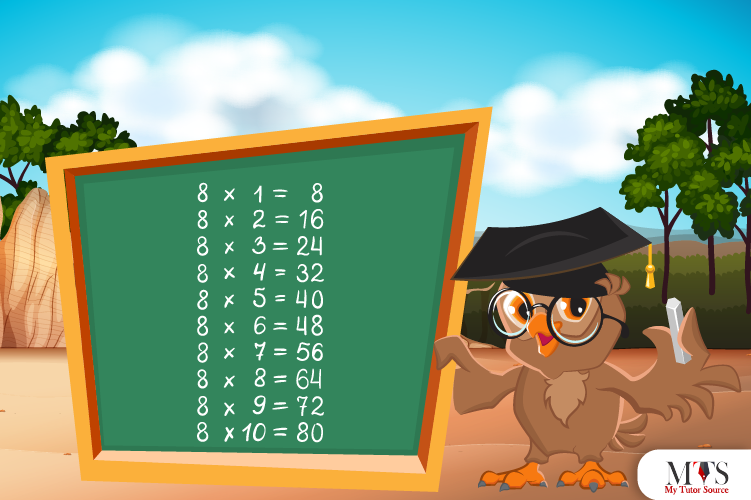## Multiplication Table of 8 – Tips to Memorize 8 Times Table & Example Questions

Among multiplication tables 1 – 20, students mostly struggle with 8 times table. They find its product confusing and intimidating as compared to other multiplication…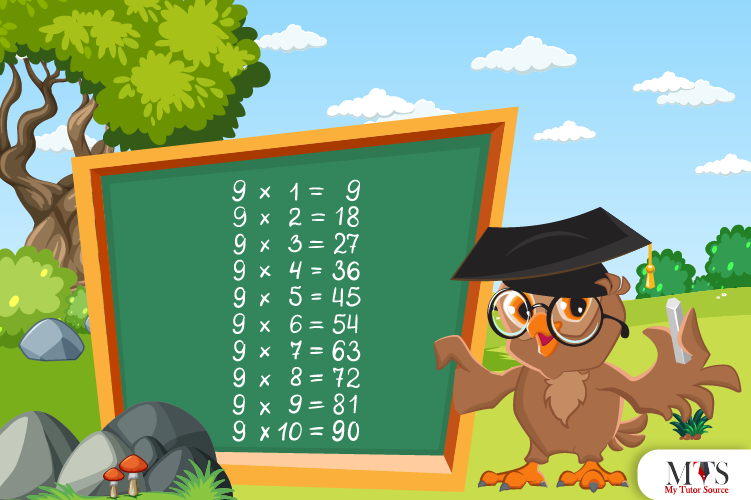## Multiplication Table of 9 – Tips to Memorize 9 Times Table & Example Questions

Students find memorizing 9 times table easier if they have already learned all lesser times tables. It is one of the easiest multiplication tables so…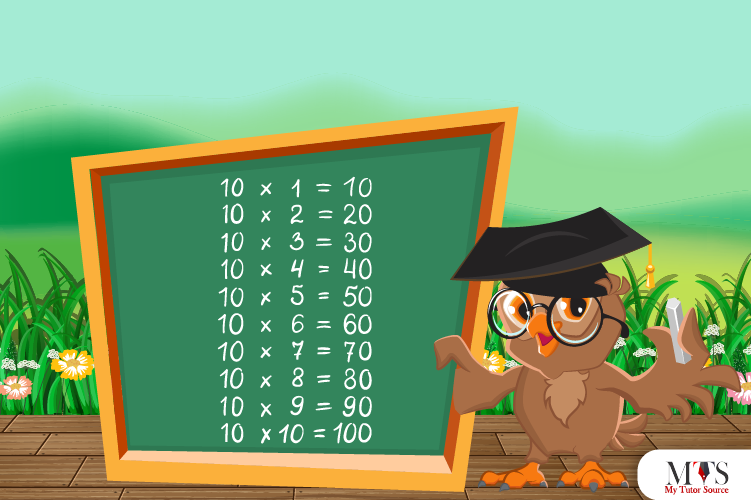## Multiplication Table of 10 – Tips to Memorize 10 Times Table & Example Questions

Here comes the multiplication table of 10 – students' favorite table so far! Teachers and math tutors encourage students to read and memorize 10 times…